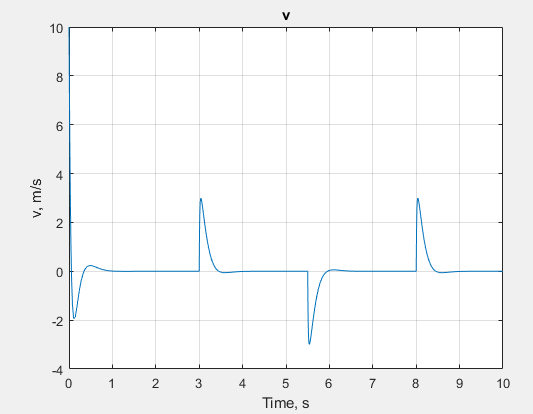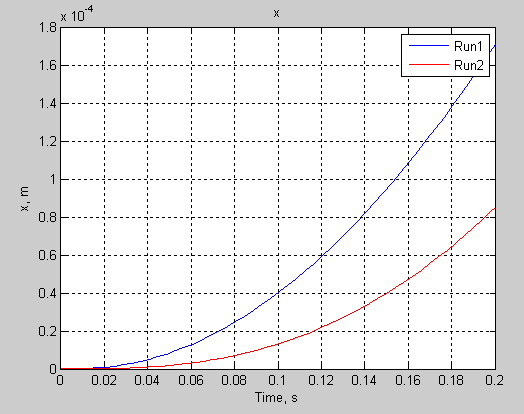# simscape.logging.plot

Plot logged simulation data for Node or Series

## Syntax

``fh = simscape.logging.plot(logobject)``
``fh = simscape.logging.plot(logobject,Name,Value)``

## Description

example

````fh = simscape.logging.plot(logobject)` plots the simulation series values along the `y`-axis, with time along the `x`-axis. `logobject` is a `simscape.logging.Node` or `simscape.logging.Series` object, or a homogeneous cell array of such objects. If `logobject` is a node, the function plots all nonempty series associated with the specified node and its children. Depending on the type of `logobj`, `fh` is a structure (for a node) or a cell array (for a series) of handles to the resulting figures. ```

example

````fh = simscape.logging.plot(logobject,Name,Value)` lets you filter the data being plotted by using one or more `Name,Value` pair arguments. For example, specify '`units`' followed by a unit name to plot only nodes and series that are commensurate with the specified unit.```

## Examples

collapse all

Plot velocity of port R of a Translational Spring block.

Open the Mass-Spring-Damper with Controller example model:

`ssc_mass_spring_damper_control`

This example model has data logging enabled for the whole model, with the Workspace variable name parameter set to `simlog_ssc_mass_spring_damper_control`.

Simulate the model to log the simulation data:

`sim('ssc_mass_spring_damper_control');`

Plot velocity of port R of the Translational Spring block `Spring`.

`simscape.logging.plot(simlog_ssc_mass_spring_damper_control.Spring.R);`Use the name-value pair arguments to filter simulation data being plotted.

For a model with the default workspace variable name, `simlog`, plot only linear positions and velocities (series that are commensurate with units of `mm` and `mm/s`), in those units, for the top-level model node, its children and their children, within the time range between 1 and 3 seconds:

`fh = simscape.logging.plot(simlog,'units',{'mm','mm/s'},'time',[1 3],'depth',2);`

Use the workspace variable name `simlog1` to log the data from the first run, and the workspace variable name `simlog2` to log the data from the second run.

Plot deformation of the Translational Spring block `TS` from both runs on the same axis, with the corresponding legend.

`simscape.logging.plot({simlog1.TS.x simlog2.TS.x},'names',{'Run1' 'Run2'});`## Input Arguments

collapse all

Simulation data to plot, specified as a `simscape.logging.Node` or `simscape.logging.Series` object, or a homogeneous cell array of such objects. `logobject` must include a full identifier path to the node or series, starting with the workspace log variable name.

The table describes the resulting plots based on the type of the `logobject` argument:

 Scalar `Series` object Plots the simulation series values along the `y`-axis, with time along the `x`-axis. Nonscalar `Series` object Plots each dimension of the series values on a different axis in the same figure window. Cell array of `Series` objects Plots all series objects with commensurate units on the same axis (superimposed), and each dimension for a nonscalar series on a different axis in the same figure window.The input arguments are binned based on commensurate units. For each bin, all `Series` objects with the same dimension as the first `Series` object in that bin are plotted and others are ignored. `Node` object Plots all nonempty series associated with the `Node` and its children (up to the level defined by the `depth`). If the `Node` has multiple simulation variable nodes as children at level 1, these children are plotted in the same figure window but on a different axis. Descendants at other levels are plotted in different figure windows. All dimensions of a nonscalar series are plotted on the same axis. Cell array of `Node` objects Plots commensurate series superimposed on the same axis.Intended for use to compare simulation data from different runs. All entries of the cell array are required to be equivalent to each other, meaning that the `Node` objects must have same hierarchy, and the series for each node must have the same dimensions and commensurate units.

### Name-Value Arguments

Specify optional pairs of arguments as `Name1=Value1,...,NameN=ValueN`, where `Name` is the argument name and `Value` is the corresponding value. Name-value arguments must appear after other arguments, but the order of the pairs does not matter.

Before R2021a, use commas to separate each name and value, and enclose `Name` in quotes.

Example: `fh = simscape.logging.plot(simlog,'units','mm','time',[1 3])` plots all of the linear position variables in the model (series that are commensurate with units of `mm`), in those units, within the time range between 1 and 3 seconds.

Number of children levels to plot for a `Node` object, specified as the comma-separated pair consisting of '`depth`' and a nonnegative integer. By default, the function plots all descendants of the `Node` object that have nonempty series. Specifying `depth` lets you limit the number of levels to plot, for example:

 `'depth',0` No children; plot the nonempty series of the specified node only. `'depth',1` Plot the nonempty series of the specified node and its children. `'depth',2` Plot the nonempty series of the specified node, its children, and their children.

If `logobject` is a `Series` object, this argument is ignored.

Example: `simscape.logging.plot(simlog.Translational_Spring,'depth',1)` plots all of the variables associated with the Translational Spring block, but not with its ports.

Plot legend, specified as the comma-separated pair consisting of '`names`' and a cell array of character vectors or string scalars. The number of elements in the cell array must be same as the number of elements of `logobject`.

By default, plots have no legend.

Time range for plotting the data, specified as the comma-separated pair consisting of '`time`' and a 1x2 vector, ```[start_time end_time]```, in seconds.

`[]` plots all data.

Units for plotting the data, specified as the comma-separated pair consisting of '`units`' and a unit name, or a cell array of unit names. Unit names must appear inside single quotes (`''`) or double quotes (`""`).

This argument plots the series values in the specified units, and also filters the data to plot only nodes and series that are commensurate with the specified unit.

Example: ```fh = simscape.logging.plot(simlog,'units',{'mm','mm/s'})``` plots all of the linear position and velocity variables in the model (series that are commensurate with units of `mm` and `mm/s`), in those units.

Alternative destination to plot the data for a `Node` object, specified as the comma-separated pair consisting of '`viewer`' and `datainspector` inside single quotes (`''`) or double quotes (`""`).

If you specify this name-value pair argument, the function plots the data in the Simulation Data Inspector. By default, the function plots the data in a plot window.

If `logobject` is a `Series` object, this argument is ignored.

## Output Arguments

collapse all

Handles to the resulting plot figure windows, returned as a structure or a cell array, depending on the type of `logobject`:

• If `logobject` is a `Node`, `fh` is a structure with the same hierarchy as the object being plotted. For example, if a specific child is not plotted then that field in the output structure is empty.

• If `logobject` is a `Series`, `fh` is a cell array.

## Version History

Introduced in R2010b